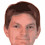## Wednesday 23 April 2014

### RGB/HSV in HLSL 5

It's been over three years since I started this thread on performing RGB/HSV conversions in HLSL. In those days, I was working with the XBox360 GPU, which has a component-wise 'max4' instruction that I took full advantage of. AMD's GCN architecture has 'min3'/'med3'/'max3' in hardware. However, the focus of GPU hardware designers seems to be shifting away from specialist SIMD pipelines. All this means that something like

`  float V = max(R, max(G, B));`

doesn't get optimised as well as you might hope.

But, given time, the Internet (or more specifically, Sam Hocevar and Emil Persson) comes up with the solution. And very elegant it is too.

HSV (and HSL) can be computed easily from HCV, where H is the hue [0..1], C is the chroma [0..1] and V is the value [0..1]. The core of a RGB-to-HCV conversion requires the RGB components to be sorted and the smallest and largest extracted:

```  float U = min(R, min(G, B));
float V = max(R, max(G, B));
float C = V - U;
```

Any fool knows that three numbers can be sorted using, at most, three comparisons. So why are we doing (effectively) four comparisons? To make things worse, we then go on to compare the largest with the three components again to determine the hue offset.

So, here is the crux of Sam and Emil's optimization:

```  float Epsilon = 1e-10;
float4 P = (G < B) ? float4(B, G, -1.0, 2.0/3.0) : float4(G, B, 0.0, -1.0/3.0);
float4 Q = (R < P.x) ? float4(P.xyw, R) : float4(R, P.yzx);
float V = Q.x;
float C = V - min(Q.w, Q.y);
float H = abs((Q.w - Q.y) / (6 * C + Epsilon) + Q.z);
```

'Q.x' contains the largest RGB component, V, and either 'Q.w' or 'Q.y' contains the smallest, U. By shuffling components of P and Q, we get the hue offset and divisor "for free." Also note the cunning use of 'Epsilon' to remove the need to check for division-by-zero. More details here.

Once we have hue/chroma/value, we can compute the HSV saturation [0..1]:

```  float S_hsv = C / (V + Epsilon);
```

Similarly, we can compute the HSL lightness [0..1] and saturation:

```  float L = V - C * 0.5;
float S_hsl = C / (1 - abs(L * 2 - 1) + Epsilon);```

In terms of speed ... a notoriously difficult thing to estimate for GPU shaders ... this new RGB-to-HCV function is no slower than the old one and faster for many configurations.

However (and here I feel a tad smug), my original implementation of HSV-to-RGB is still faster than Sam's alternative. Though I'm sure that with a particularly aggressive optimizer on a more scalar configuration, there'd be hardly anything in it.

```  float R = abs(H * 6 - 3) - 1;
float G = 2 - abs(H * 6 - 2);
float B = 2 - abs(H * 6 - 4);
float3 RGB = ((saturate(float3(R,G,B)) - 1) * S + 1) * V;
```

I've placed the HLSL snippets on my main website; I hope no typos have crept in like last time.

For completeness, I've left in the HCY and HCL conversions.

1.Great work!

I have a query for you. I am looking to manipulate only the v channel of an image and do not need to touch the h and s channels. So I only need the h and s to get back to RGB after modifying the v. Is there a way in this case to save computation by not fully computing h and s and instead only compute enough information which can be combined with the updated v value to get back to RGB?

2.Hi Anonymous,

Yes, you can do that ... though there's a caveat. I'm assuming you're talking about the 'V' channel in HSV space; in which case, V = max(R,G,B). So to modify the RGB components you simply have to scale R, G and B by a common amount. Supposing your input float3 is "rgb_in" and your required 'V' is "v_out", then:

float v_in = max(max(rgb_in.r, rgb_in.g), rgb_in.b);
float scale = v_out / v_in;
float3 rgb_out = rgb_in * scale;

The problem comes when the input is fully black, i.e. "v_in" is zero. One solution could be to produce an appropriately-bright grey value based on "v_out" under those circumstances:

float v_in = max(max(rgb_in.r, rgb_in.g), rgb_in.b);
float3 rgb_out;
if (v_in < 1e-10) {
rgb_out = float3(v_out, v_out, v_out);
} else {
float scale = v_out / v_in;
rgb_out = rgb_in * scale;
}

I hope this helps.

3.Having said all that, actually setting all values of 'V' throughout an image to a fixed value is a strange thing to do. If you want to change the "brightness" then linear scaling the RGB channels by an amount makes sense. Of course, if you want to increase the brightness (i.e. the scaling factor is > 1) just make sure you clamp the result.

Alternatively, you may be wanting to set the 'V' without changing the 'H' or the 'S'. This can be a little bit more tricky. I'll add another entry for that topic.

4.This comment has been removed by the author.